EnchantedLearning.com is a user-supported site.
As a bonus, site members have access to a banner-ad-free version of the site, with print-friendly pages.

The following printouts are of simple addition worksheets.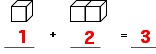Adding Groups of CubesAdd two groups of cubes.Adding Groups of ItemsAdd two groups of objects, including stars, triangles, octagons, etc.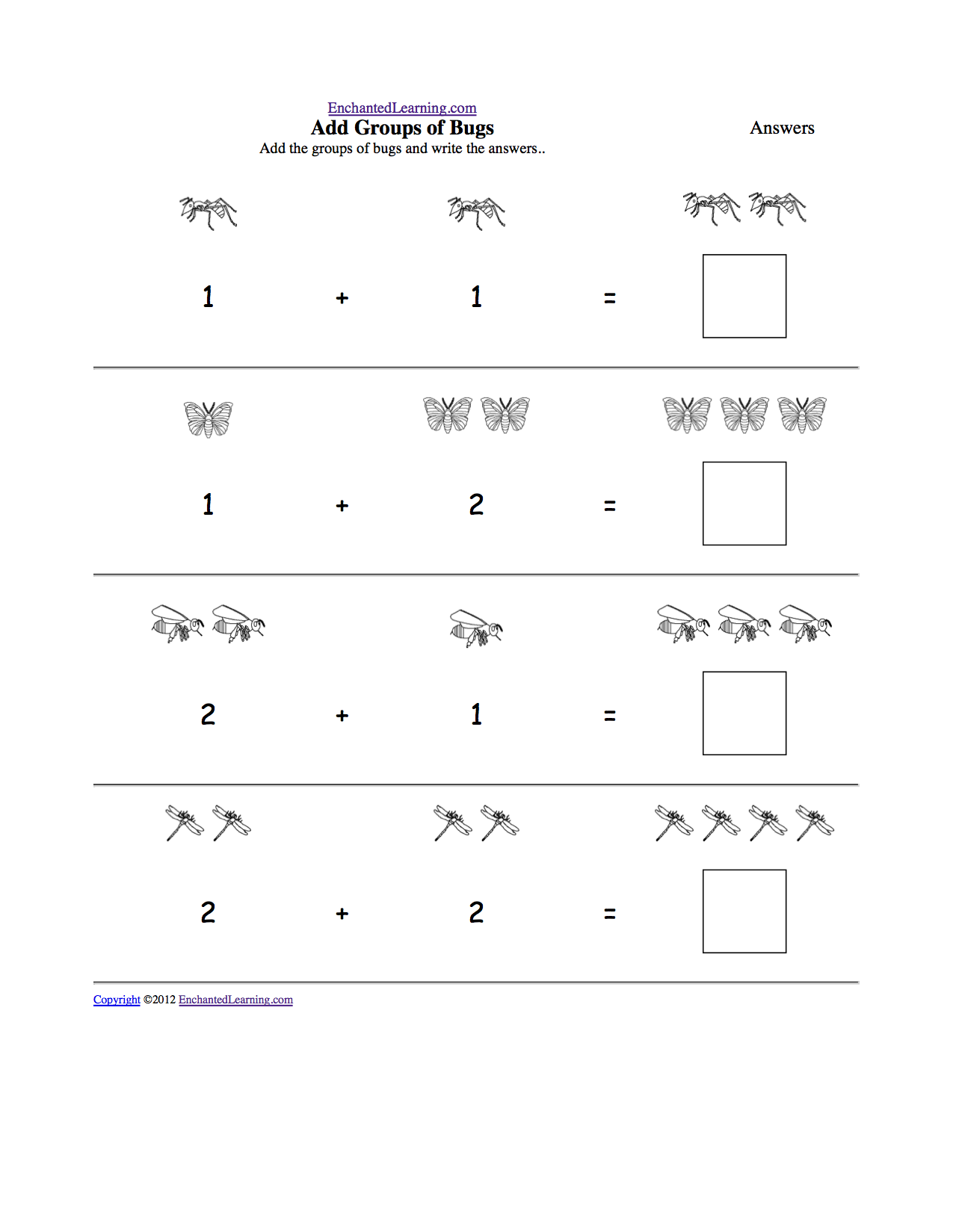Add Groups of ItemsPrintable Worksheet Add the groups of common items and write the answers.Dinosaur Math Coloring ActivitiesDo simple addition problems to color a dinosaur scene. For beginning readers in grades 1 and 2. 2, 4, 6, 8, 10, ...Skip CountingPrintablesSkip counting is good addition practice and a great introduction to multiplication. Count by 2s, 3s, s, and so on in these worksheets.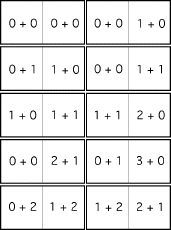Printable Set of Addition DominoesPrint a set of subtraction dominoes; each tile has addition problems on it (for example, 0 + 5). The numbers from zero to six are used in the addition problems and the answers are the numbers from zero to six. In this game the student matches the answers of addition problems.Printable Set of Addition Dominoes #2Print a set of addition dominoes; each tile has addition problems on it (for example, 4 + 7). The numbers from one to nine are used in the addition problems and the answers are the numbers from seven to thirteen. In this game the student matches the answers of addition problems. 3 + 4 = Adding Strings of 2 NumbersAdd horizontal strings of two numbers. 2 + 4 + 6 = Adding Strings of 3 NumbersAdd horizontal strings of three numbers. Tip: add groups that sum to ten first. 2 + 4 + 6 + 3 = Adding Strings of 4 NumbersAdd horizontal strings of four numbers. Tip: add groups that sum to ten first. 8 + 5 + 8 + 1 + 1 = Adding Strings of Many NumbersAdd horizontal strings of four numbers. Tip: add groups that sum to ten first.Addition Follow-the-Arrows Puzzles Do addition problems along the paths according to the arrows until you get to the end. You should get to the same final answer three different ways. Puzzle #1, or the answers Puzzle #2, or the answers Puzzle #3, or the answers Puzzle #4, or the answers Puzzle #5, or the answers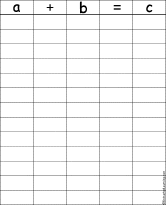Addition ChartWrite many equations in which a+b=c, like 3+1=4.Add, then Decode the Secret MessagesIn these theme-based addition puzzles, the student solves addition problems, then use an alphabet code to decode a secret message.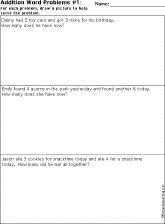Word Problems: Adding 2 NumbersDraw a picture to help you solve each problem.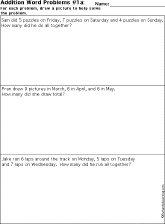Word Problems: Adding 3 NumbersDraw a picture to help you solve each problem.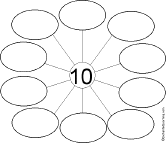Many Ways of Expressing NumbersMath Graphic Organizers10 graphic organizers to use to express numbers in different ways, from "2 Ways to Make 2" to "10 Ways to Make 10." 1 Digit Plus 1 DigitAdd two one-digit numbers in a grid.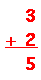1 Digit Plus 1 DigitAdd two one-digit numbers - vertical orientation.2 Digits Plus 1 DigitAdd a two-digit number plus a one-digit number - vertical orientation.2 Digits Plus 2 DigitsAdd a two-digit number plus a two-digit number - vertical orientation.3 Digits Plus 2 DigitsAdd a three-digit number plus a two-digit number - vertical orientation.3 Digits Plus 3 DigitsAdd a three-digit number plus a three-digit number - vertical orientation.Addition: Find the Missing Digit1 Digit Plus 1 DigitFind the missing digit in addition problems (adding one digit plus one digit numbers).Addition: Find the Missing Digit2 Digits Plus 1 DigitFind the missing digit in addition problems (adding two digit plus one digit numbers).Addition: Find the Missing Digit2 Digits Plus 2 DigitsFind the missing digit in addition problems (adding two digit plus two digit numbers).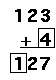Addition: Find the Missing Digit3 Digits Plus 1 DigitFind the missing digit in addition problems (adding three digit plus one digit numbers).Addition: Find the Missing Digit3 Digits Plus 2 DigitsFind the missing digit in addition problems (adding three digit plus two digit numbers).Addition: Find the Missing Digit3 Digits Plus 3 DigitsFind the missing digit in addition problems (adding three digit plus two digit numbers).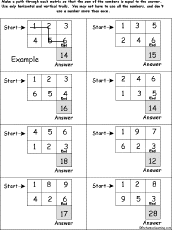Addition Path-o-Math Puzzles: 2 by 3Make a path through each number matrix so that the sum of the numbers is equal to the given answer. Use only horizontal and vertical trails. You may not have to use all the numbers, and don't use a number more than once. Puzzle #1, or the answers Puzzle #2, or the answers Puzzle #3, or the answers Puzzle #4, or the answers Puzzle #5, or the answers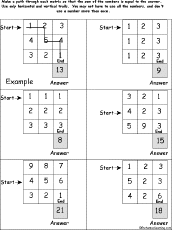Addition Path-o-Math Puzzles: 3 by 3Make a path through each number matrix so that the sum of the numbers is equal to the given answer. Use only horizontal and vertical trails. You may not have to use all the numbers, and don't use a number more than once. Puzzle #1, or the answers Puzzle #2, or the answers Puzzle #3, or the answers Puzzle #4, or the answers Puzzle #5, or the answers

 Adding Decimals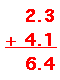Each worksheet has decimal numbers to add.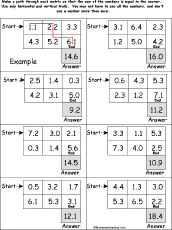Adding Decimals Path-o-Math Puzzles: 2 by 3Make a path through each number matrix so that the sum of the decimals is equal to the given answer. Use only horizontal and vertical trails. You may not have to use all the numbers, and don't use a number more than once. Puzzle #1, or the answers Puzzle #2, or the answers Puzzle #3, or the answers Puzzle #4, or the answers Puzzle #5, or the answers

 Adding Fractions With the Same Denominator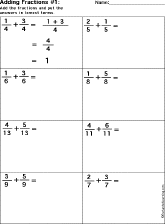Add the fractions (they have the denominators). Adding Fractions With Different DenominatorsAdd the fractions (they have different denominators).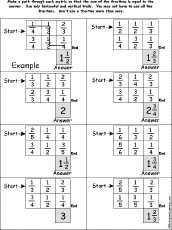Adding Fractions Path-o-Math Puzzles: 2 by 3Make a path through each number matrix so that the sum of the fractions is equal to the given answer. Use only horizontal and vertical trails. You may not have to use all the numbers, and don't use a number more than once. Puzzle #1, or the answers Puzzle #2, or the answers Puzzle #3, or the answers Puzzle #4, or the answers Puzzle #5, or the answers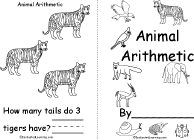Animal Arithmetic (with pictures)A Printable Activity BookA short, printable book about addition for early readers. The book has pages for the student to cut out, questions to read, pictures to help solve the questions (like, "How many legs do 2 ladybugs have?"), and objects to count. The pages include: tiger tails, eagle eyes, toad tongues, bird beaks, wasp wings, fox feet, snail shells, elephant ears, shark snouts, ladybug legs, antelope antlers, ant antennae, and moose mouths.Animal Arithmetic (no pictures)A Printable Activity BookA short, printable book about addition for early readers. The book has pages for the student to cut out, questions to read, pictures to draw in order to solve the questions (like, "How many paws do 3 cats have?"), and objects to count. The pages include: bird wings, elephant trunks, dog eyes, spider legs, monkey tails, rabbit ears, octopus arms, bull horns, people toes, cat paws, and frog mouths.Counting CoinsA Printable Activity BookA short, printable book about coins for early readers. Color and count the pennies, nickels, dimes, and quarters, then tally up what they are worth.Firefighting AdditionA Printable Activity BookA short, printable book about addition. This activity book asks the student to solve simple addition problems stated in terms of firefighting words, including firetrucks, firefighters, axes, helmets, dogs, hoses, hydrants, boots, and fires put out.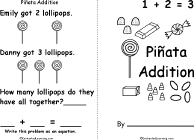Piñata AdditionA Printable Activity BookA short, printable book about addition. This activity book asks the student to solve simple addition problems stated in terms of candies, including lollipops, candy bars, gumdrops, candy corn, jelly beans, caramels, mints, chocolate bars, and licorice sticks.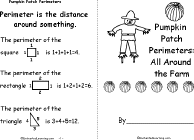Pumpkin Patch Perimeters: All Around the FarmPrintable BookA short, printable book about perimeters, using pumpkin patch terms, including the perimeters of a pumpkin patch, chicken coop, pigpen, corn field, goat pen, horse corral, barnyard, and vegetable garden.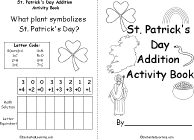St. Patrick's Day Addition Activity BookA St. Patrick's Day addition activity book in which the readers solves a series of addition problems and then does a letter substitution in order to answer St. Patrick's Day questions.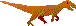Dinosaur Math-Code PuzzlesDo simple addition or subtraction problems and letter substitutions to answer dinosaur questions. (Grade 1 and 2).More math-code activities.Shark Math-Code PuzzlesThe student does simple math problems and then does letter substitutions to answer a shark question. (Grade 1)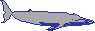Whale Math-Code PuzzlesThe student does simple math problems and then does letter substitutions to answer a whale-related question. (Grade 1)St. Patrick's Day Addition Activity BookA St. Patrick's Day addition activity book in which the readers solves a series of addition problems and then does a letter substitution in order to answer St. Patrick's Day questions.

File Folder Games on Adding Numbers:Add the Numbers #1: File Folder GameThis is a simple game that can be made from a manila file folder. In this game, the student adds two one-digit numbers, and then checks the answer by opening a paper window under the math problem. This is an activity that stores easily!Add the Numbers #2: File Folder GameThis is a simple game that can be made from a manila file folder. In this game, the student adds two one-digit numbers, and then checks the answer by opening a paper window under the math problem. This is an activity that stores easily!

Enchanted Learning®
Over 35,000 Web Pages
Sample Pages for Prospective Subscribers, or click below

 Overview of Site What's New Enchanted Learning Home Monthly Activity Calendar Books to Print Site Index K-3 Crafts K-3 Themes Little ExplorersPicture dictionary PreK/K Activities Rebus Rhymes Stories Writing Cloze Activities Essay Topics Newspaper Writing Activities Parts of Speech Fiction The Test of Time Biology Animal Printouts Biology Label Printouts Biomes Birds Butterflies Dinosaurs Food Chain Human Anatomy Mammals Plants Rainforests Sharks Whales Physical Sciences: K-12 Astronomy The Earth Geology Hurricanes Landforms Oceans Tsunami Volcano Languages Dutch French German Italian Japanese (Romaji) Portuguese Spanish Swedish Geography/History Explorers Flags Geography Inventors US History Other Topics Art and Artists Calendars College Finder Crafts Graphic Organizers Label Me! Printouts Math Music Word Wheels

## Enchanted Learning Search

 Search the Enchanted Learning website for: